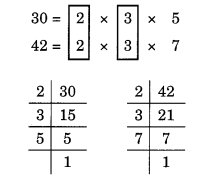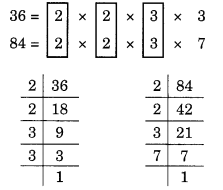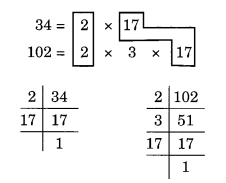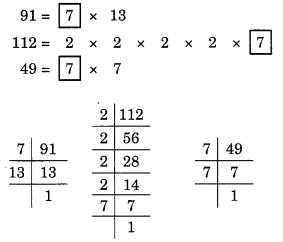# NCERT Solutions For Class 6 Maths Playing With Numbers Exercise 3.6

ncert textbook

## NCERT Solutions For Class 6 Maths Playing With Numbers Exercise 3.6

NCERT Solutions for Class 6 Maths Chapter 3 Playing With Numbers Ex 3.6

Exercise 3.6

Question 1.
Find the HCF of the following numbers:
(a) 18, 48
(b) 30, 42
(c) 18, 60
(d) 27,63
(e) 36,84
(f) 34, 102
(g) 70, 105, 175
(h) 91, 112, 49
(i) 18, 54, 81
(j) 12, 45, 75
Solution:
(a) Given numbers are 18 and 48.
Prime factorisations of 18 and 48 are:Here, the common factors are 2 and 3.
Hence, the HCF = 2 x 3 = 6.

(b) The given numbers are 30 and 42.
Prime factorisations of 30 and 42, are:Here, the common factors are 2 and 3.
Hence, the HCF = 2 x 3 = 6.

(c) Given numbers are 18 and 60.
Prime factorisations of 18 and 60 are:Here, the common factors are 2 and 3.
Hence, the HCF of 18 and 60 = 2 x 3 = 6.

(d) Given numbers are 27 and 63.
Prime factorisations of 27 and 63 are:Here, the common factor is 3 (occurring twice).
Hence, the HCF = 3 x 3 = 9.

(e) Given numbers are 36 and 84.
Prime factorisations of 36 and 84 are:Here, the common factors are 2, 2 and 3.
Hence, the HCF = 2 x 2 x 3 = 12.

(f) Given numbers are 34 and 102.
Prime factorisations of 34 and 102 are:Here, the common factors are 2 and 17.
Thus, HCF is 2 x 17 = 34.

(g) The given numbers are 70, 105 and 175.
Prime factorisatios of 70, 105 and 175 are:Here, common factors are 5 and 7.
Hence, the HCF of 70, 105 and 175 is 5 x 7 = 35.

(h) Given numbers are 91, 112 and 49.
Prime factorisations of 91, 112 and 49 are:Here, the common factor is 7.
Hence, the HCF = 7.

(i) Given numbers are 18, 54 and 81.
Prime factorisations of 18, 54 and 81 are:Here, the common factor is 3 (occurring twice).
Thus, the HCF = 3 x 3 = 9.

(j) Given numbers are 12, 45 and 75.
Prime factorisations of 12, 45 and 75 are:Here, the common factor is 3.
Hence, the HCF = 3.

Question 2.
What is the HCF of two consecutive
(a) numbers?
(b) even numbers?
(c) odd numbers?
Solution:
(a) The common factor of two consecutive numbers is always 1.
Hence, the HCF = 1.
(b) The common factors of two consecutive even numbers are 1 and 2.
Hence, the HCF = 1 x 2 = 2.
(c) The common factor of two consecutive odd numbers is 1.
Hence, the HCF = 1.

Question 3.
HCF of co-prime numbers 4 and 15 was found as follows by factorisation:
4 = 2 x 2 and 15 = 3 x 15. Since there is no common prime factors, so HCF of 4 and 15 is 0.
Is the answer correct? If not, what is the correct HCF?
Solution: